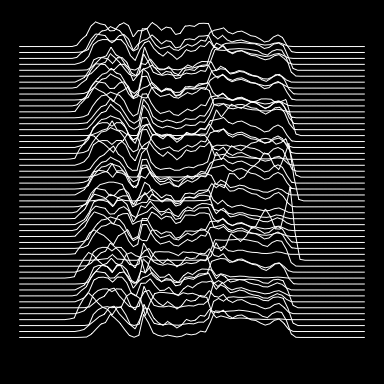# 3. calculations

The rintcal package also provides some functions related to radiocarbon calibration. First there are two functions to calculate radiocarbon ages from pMC values (in this case of a postbomb date):

pMC.age(150, 1)
##  -3257    53

and the other way round:

age.pMC(-2300, 40)
##           y    sdev
## [1,] 133.15 0.66138

The same for calculations in the F14C realm:

F14C.age(.150, .01)
##          y   sdev
## [1,] 15240 518.44

and the other way round:

age.F14C(-2300, 40)
##           y      sdev
## [1,] 1.3315 0.0066138

To transfer $$\Delta^{14}C$$ (a proxy for atmospheric 14C concentration at t cal BP) to F14C, and the other way around:

F14C.D14C(0.71, t=4000)
##  151.8406
D14C.F14C(152, 4000)
##  0.7100983

These functions can be used to investigate $$\Delta^{14}C$$ over time:

cc <- ccurve()
cc.Fmin <- age.F14C(cc[,2]+cc[,3])
cc.Fmax <- age.F14C(cc[,2]-cc[,3])
cc.D14Cmin <- F14C.D14C(cc.Fmin, cc[,1])
cc.D14Cmax <- F14C.D14C(cc.Fmax, cc[,1])
par(mar=c(4,3,1,3), bty="l")
plot(cc[,1]/1e3, cc.D14Cmax, type="l", xlab="kcal BP", ylab="")
mtext(expression(paste(Delta, ""^{14}, "C")), 2, 1.7)
lines(cc[,1]/1e3, cc.D14Cmin)
par(new=TRUE)
plot(cc[,1]/1e3, (cc[,2]+cc[,3])/1e3, type="l", xaxt="n", yaxt="n", col=4, xlab="", ylab="")
lines(cc[,1]/1e3, (cc[,2]-cc[,3])/1e3, col=4)
axis(4, col=4, col.axis=4)
mtext(expression(paste(""^{14}, "C kBP")), 4, 2, col=4)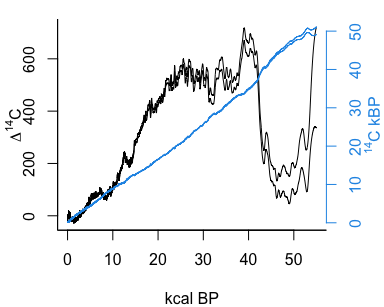The above functions can be used to calculate the effect of contamination on radiocarbon ages, e.g. what age would be observed if material with a “true” radiocarbon age of 5000 +- 20 14C BP would be contaminated with 1% of modern carbon (F14C=1)?

contaminate(5000, 20, .01, 1)
##           y   sdev
## [1,] 4930.9 19.779

The effect of different levels of contamination can also be visualised:

real.14C <- seq(0, 50e3, length=200)
contam <- seq(0, .1, length=101) # 0 to 10% contamination
contam.col <- rainbow(length(contam))
plot(0, type="n", xlim=c(0, 55e3), xlab="real 14C age", ylim=range(real.14C), ylab="observed 14C age")
for(i in 1:length(contam))
lines(real.14C, contaminate(real.14C, c(), contam[i], 1, decimals=5), col=contam.col[i])
contam.legend <- seq(0, .1, length=6)
contam.col <- rainbow(length(contam.legend)-1)
text(50e3, contaminate(50e3, c(), contam.legend, 1),
labels=contam.legend, col=contam.col, cex=.7, offset=0, adj=c(0,.8))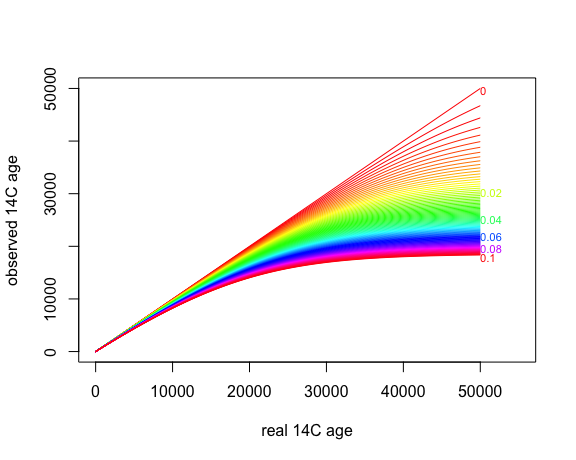If that is too much code for you, try this function instead:

draw.contamination()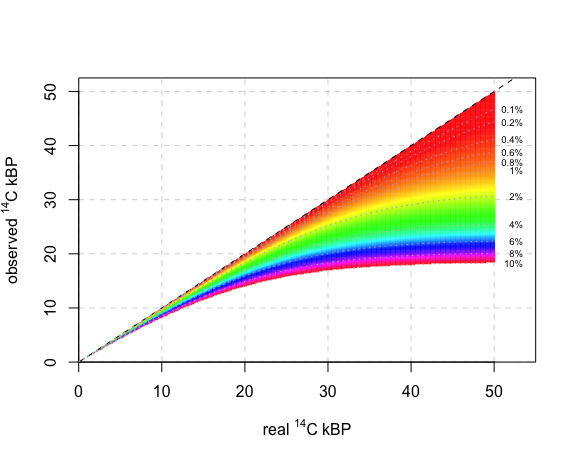Now on to calibration. We can obtain the calibrated probability distributions from radiocarbon dates, e.g., one of 130 +- 20 C14 BP:

calib.130 <- caldist(130, 20, BCAD=TRUE)
plot(calib.130, type="l")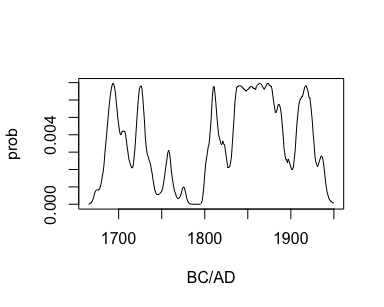For reporting purposes, calibrated dates are often reduced to their 95% highest posterior density (hpd) ranges (please report all, not just your favourite one!):

hpd(calib.130)
##      from   to perc
## [1,] 1683 1738 24.4
## [2,] 1755 1761  1.8
## [3,] 1801 1938 68.6

Additionally, calibrated dates are often reduced to single point estimates. Note however how poor representations they are of the entire calibrated distribution!

calib.2450 <- caldist(2450, 20)
plot(calib.2450, type="l")
points.2450 <- point.estimates(calib.2450)
points.2450
## weighted mean        median          mode      midpoint
##        2539.9        2512.3        2666.0        2531.5
abline(v=points.2450, col=1:4, lty=2)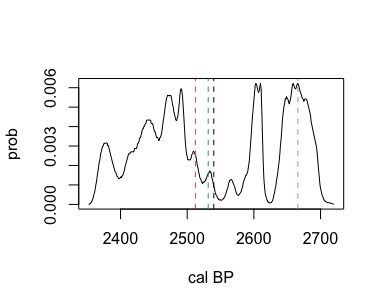Want a plot of the radiocarbon and calibrated distributions, together with their hpd ranges?

calibrate(130,20)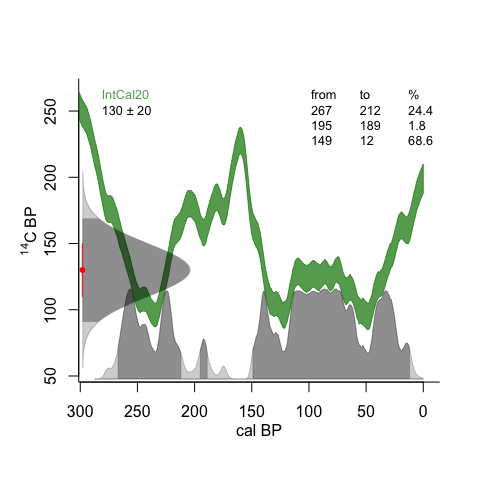You can also draw one or more calibrated distributions:

set.seed(123)
dates <- sort(sample(500:2500,5))
errors <- .05*dates
depths <- 1:length(dates)
my.labels <- c("my", "very", "own", "simulated", "dates")
draw.dates(dates, errors, depths, BCAD=TRUE, labels=my.labels, age.lim=c(0, 1800))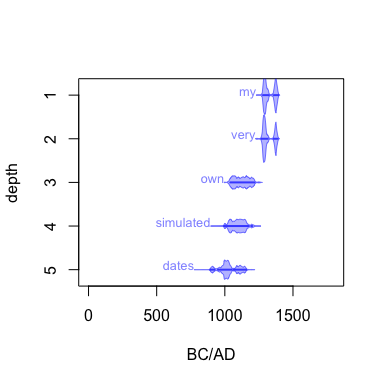or add them to an existing plot:

plot(300*1:5, 1:5, xlim=c(0, 1800), ylim=c(5,0), xlab="AD", ylab="dates")
draw.dates(dates, errors, depths, BCAD=TRUE, add=TRUE, labels=my.labels, mirror=FALSE)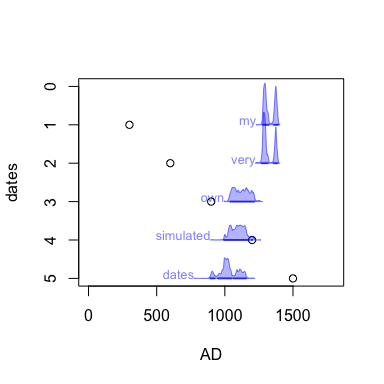or get creative (inspired by Jocelyn Bell Burnell, Joy Division and the Hallstatt Plateau):

par(bg="black", mar=rep(1, 4))
n <- 50; set.seed(1)
draw.dates(rnorm(n, 2450, 30), rep(25, n), n:1,
mirror=FALSE, draw.base=FALSE, draw.hpd=FALSE, col="white",
threshold=1e-28, age.lim=c(2250, 2800), ex=.8)